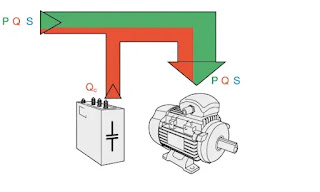# Power Factor Correction techniques

0
597

## What is Power Factor Correction?

### Why Power Factor Correction?

#### So without power factor correction, to achieve the required output of 1.725 kW (7.5 A) we have to supply a power of 2.3 kVA (10 A) to the motor. Also current of 10 A is flowing in the circuit but only 7.5 A of that current is producing useful output. Hence burden on system and also utility bill increases due to poor power factor.### Power Factor Correction Formula

• #### So it is simply that if we use a capacitor in parallel to draw the leading current we can cancel the effect of lagging inductive current. Hence we can improve the power factor of the circuit.### 1. Power factor correction by using a capacitor bank#### Here Star and Delta connected capacitor bank is shown in the figure.### 3. Power factor correction by Phase Advancer

• #### Another attractive feature of phase advancer is that if we supply more amp-turns than needed, the motor will operate in an overexcited state (at leading PF).

READ HERE  Power Factor Improvement Methods